# 1 零长度数组概念

``````struct Packet
{
int state;
int len;
char cData; //这里的0长结构体就为变长结构体提供了非常好的支持
};``````

• 用途 : 长度为0的数组的主要用途是为了满足需要变长度的结构体

• 用法 : 在一个结构体的最后, 申明一个长度为0的数组, 就可以使得这个结构体是可变长的. 对于编译器来说, 此时长度为0的数组并不占用空间, 因为数组名本身不占空间, 它只是一个偏移量, 数组名这个符号本身代表了一个不可修改的地址常量

(注意 : 数组名永远都不会是指针!), 但对于这个数组的大小, 我们可以进行动态分配

# 2 0长度数组的用途

• 定长数据缓冲区, 设置一个足够大小 `MAX_LENGTH` 的数据缓冲区

• 设置一个指向实际数据的指针, 每次使用时, 按照数据的长度动态的开辟数据缓冲区的空间.

## 2.1 定长包(开辟空间, 释放, 访问)

• 数据结构定义
``````//  定长缓冲区
struct max_buffer
{
int     len;
char    data[MAX_LENGTH];
};``````
• 数据结构大小

• 数据包的构造

``````    ///  开辟
if ((mbuffer = (struct max_buffer *)malloc(sizeof(struct max_buffer))) != NULL)
{
mbuffer->len = CURR_LENGTH;
memcpy(mbuffer->data, "Hello World", CURR_LENGTH);

printf("%d, %s\n", mbuffer->len, mbuffer->data);
}``````
• 访问

• 释放

``````    /// 销毁
free(mbuffer);
mbuffer = NULL;``````
• 小结

1. 使用定长数组, 作为数据缓冲区, 为了避免造成缓冲区溢出, 数组的大小一般设为足够的空间 `MAX_LENGTH`, 而实际使用过程中, 达到 `MAX_LENGTH` 长度的数据很少, 那么多数情况下, 缓冲区的大部分空间都是浪费掉的.

2. 但是使用过程很简单, 数据空间的开辟和释放简单, 无需程序员考虑额外的操作

## 2.2 指针数据包(开辟空间, 释放, 访问)

• 数据包定义
``````struct point_buffer
{
int     len;
char    *data;
};``````
• 数据结构大小

• 空间分配

``````    // =====================
// 指针数组  占用-开辟-销毁
// =====================
///  占用
printf("the length of struct test3:%d\n",sizeof(struct point_buffer));
///  开辟
if ((pbuffer = (struct point_buffer *)malloc(sizeof(struct point_buffer))) != NULL)
{
pbuffer->len = CURR_LENGTH;
if ((pbuffer->data = (char *)malloc(sizeof(char) * CURR_LENGTH)) != NULL)
{
memcpy(pbuffer->data, "Hello World", CURR_LENGTH);

printf("%d, %s\n", pbuffer->len, pbuffer->data);
}
}``````
1. 首先, 需为结构体分配一块内存空间;

2. 其次再为结构体中的成员变量分配内存空间.

• 释放

``````    /// 销毁
free(pbuffer->data);
free(pbuffer);
pbuffer = NULL;``````
• 小结

1. 使用指针结果作为缓冲区, 只多使用了一个指针大小的空间, 无需使用 `MAX_LENGTH` 长度的数组, 不会造成空间的大量浪费.

2. 但那是开辟空间时, 需要额外开辟数据域的空间, 施放时候也需要显示释放数据域的空间, 但是实际使用过程中, 往往在函数中开辟空间, 然后返回给使用者指向 `struct point_buffer` 的指针, 这时候我们并不能假定使用者了解我们开辟的细节, 并按照约定的操作释放空间, 因此使用起来多有不便, 甚至造成内存泄漏

## 2.3 变长数据缓冲区(开辟空间, 释放, 访问)

`GNU C` 的0长度数组, 也叫变长数组, 柔性数组就是这样一个扩展. 对于0长数组的这个特点，很容易构造出变成结构体，如缓冲区，数据包等等：

• 数据结构定义
``````//  0长度数组
struct zero_buffer
{
int     len;
char    data;
};``````
• 数据结构大小

• 开辟空间

``````    ///  开辟
if ((zbuffer = (struct zero_buffer *)malloc(sizeof(struct zero_buffer) + sizeof(char) * CURR_LENGTH)) != NULL)
{
zbuffer->len = CURR_LENGTH;
memcpy(zbuffer->data, "Hello World", CURR_LENGTH);

printf("%d, %s\n", zbuffer->len, zbuffer->data);
}``````
• 释放空间

``````    ///  销毁
free(zbuffer);
zbuffer = NULL;``````

## 2.4 总结

``````// zero_length_array.c
#include <stdio.h>
#include <stdlib.h>

#define MAX_LENGTH      1024
#define CURR_LENGTH      512

//  0长度数组
struct zero_buffer
{
int     len;
char    data;
}__attribute((packed));

//  定长数组
struct max_buffer
{
int     len;
char    data[MAX_LENGTH];
}__attribute((packed));

//  指针数组
struct point_buffer
{
int     len;
char    *data;
}__attribute((packed));

int main(void)
{
struct zero_buffer  *zbuffer = NULL;
struct max_buffer   *mbuffer = NULL;
struct point_buffer *pbuffer = NULL;

// =====================
// 0长度数组  占用-开辟-销毁
// =====================
///  占用
printf("the length of struct test1:%d\n",sizeof(struct zero_buffer));
///  开辟
if ((zbuffer = (struct zero_buffer *)malloc(sizeof(struct zero_buffer) + sizeof(char) * CURR_LENGTH)) != NULL)
{
zbuffer->len = CURR_LENGTH;
memcpy(zbuffer->data, "Hello World", CURR_LENGTH);

printf("%d, %s\n", zbuffer->len, zbuffer->data);
}
///  销毁
free(zbuffer);
zbuffer = NULL;

// =====================
// 定长数组  占用-开辟-销毁
// =====================
///  占用
printf("the length of struct test2:%d\n",sizeof(struct max_buffer));
///  开辟
if ((mbuffer = (struct max_buffer *)malloc(sizeof(struct max_buffer))) != NULL)
{
mbuffer->len = CURR_LENGTH;
memcpy(mbuffer->data, "Hello World", CURR_LENGTH);

printf("%d, %s\n", mbuffer->len, mbuffer->data);
}
/// 销毁
free(mbuffer);
mbuffer = NULL;

// =====================
// 指针数组  占用-开辟-销毁
// =====================
///  占用
printf("the length of struct test3:%d\n",sizeof(struct point_buffer));
///  开辟
if ((pbuffer = (struct point_buffer *)malloc(sizeof(struct point_buffer))) != NULL)
{
pbuffer->len = CURR_LENGTH;
if ((pbuffer->data = (char *)malloc(sizeof(char) * CURR_LENGTH)) != NULL)
{
memcpy(pbuffer->data, "Hello World", CURR_LENGTH);

printf("%d, %s\n", pbuffer->len, pbuffer->data);
}
}
/// 销毁
free(pbuffer->data);
free(pbuffer);
pbuffer = NULL;

return EXIT_SUCCESS;
}``````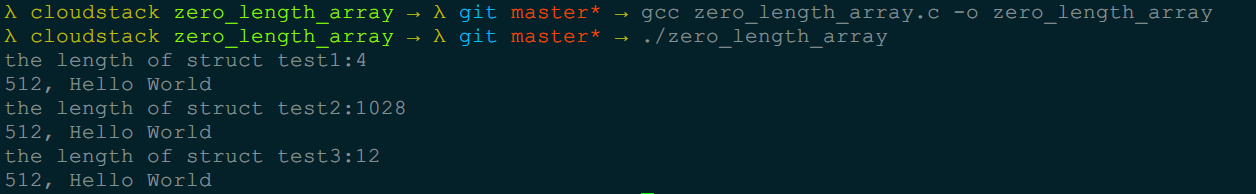• 长度为0的数组并不占有内存空间, 而指针方式需要占用内存空间.

• 对于长度为0数组, 在申请内存空间时, 采用一次性分配的原则进行; 对于包含指针的结构体, 才申请空间时需分别进行, 释放时也需分别释放.

• 对于长度为的数组的访问可采用数组方式进行

# 3 GNU Document中 变长数组的支持

6.17 Arrays of Length Zero

C Struct Hack – Structure with variable length array

1、`-pedantic` 选项，那么使用了扩展语法的地方将产生相应的警告信息

2、`-Wall` 使用它能够使GCC产生尽可能多的警告信息

3、`-Werror`, 它要求GCC将所有的警告当成错误进行处理

``````// 1.c
#include <stdio.h>
#include <stdlib.h>

int main(void)
{
char a;
printf("%ld", sizeof(a));
return EXIT_SUCCESS;
}``````

``````gcc 1.c -Wall   # 显示所有警告
#none warning and error

gcc 1.c -Wall -pedantic  # 对GNU C的扩展显示警告
1.c: In function ‘main’:
1.c:7: warning: ISO C forbids zero-size array ‘a’

gcc 1.c -Werror -Wall -pedantic # 显示所有警告同时GNU C的扩展显示警告, 将警告用error显示
cc1: warnings being treated as errors
1.c: In function ‘main’:
1.c:7: error: ISO C forbids zero-size array ‘a’``````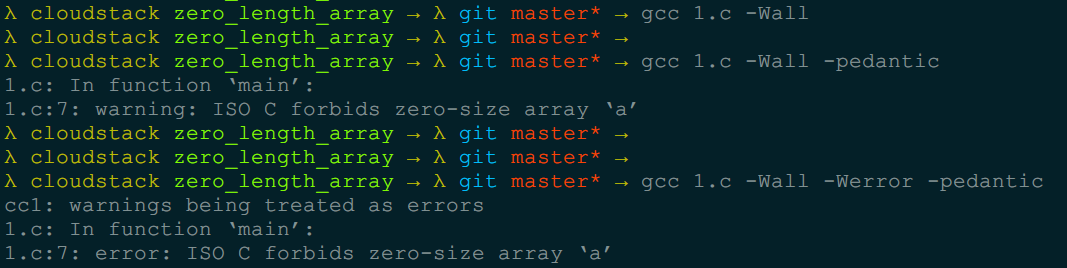0长度数组其实就是灵活的运用的数组指向的是其后面的连续的内存空间

``````struct buffer
{
int     len;
char    data;
};``````

• 定长数组定义了一个足够大的缓冲区, 这样使用方便, 但是每次都造成空间的浪费
• 指针的方式, 要求程序员在释放空间是必须进行多次的free操作, 而我们在使用的过程中往往在函数中返回了指向缓冲区的指针, 我们并不能保证每个人都理解并遵从我们的释放方式

`C99`之后，也加了类似的扩展，只不过用的是 `char payload[]`这种形式（所以如果你在编译的时候确实需要用到`-pedantic`参数，那么你可以将`char payload`类型改成`char payload[]`, 这样就可以编译通过了，当然你的编译器必须支持C99标准的，如果太古老的编译器，那可能不支持了)

``````// 2.c payload
#include <stdio.h>
#include <stdlib.h>

{
int   len;
char  data[];
};

int main(void)
{
printf("%ld", sizeof(pay));
return EXIT_SUCCESS;
}``````

``gcc 2.c -pedantic -std=c99``
• 1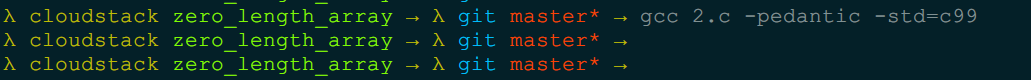GNU手册还提供了另外两个结构体来说明，更容易看懂意思：

``````struct f1 {
int x;
int y[];
} f1 = { 1, { 2, 3, 4 } };

struct f2 {
struct f1 f1;
int data;
} f2 = { { 1 }, { 5, 6, 7 } };``````

``````f1.x = 1
f1.y = 2
f1.y = 3
f1.y = 4``````

``````f2.f1.x = 1
f2.f1.y = 5
f2.f1.y = 6
f2.f1.y = 7``````

``````main.c:37:9: error: flexible array member not at end of struct
int y[];
^``````

``````struct f3 {
int x;
int *y;
};``````

``````// 3.c
#include <stdio.h>
#include <stdlib.h>

struct f1 {
int x;
int y[];
} f1 = { 1, { 2, 3, 4 } };

struct f2 {
struct f1 f1;
int data;
} f2 = { { 1 }, { 5, 6, 7 } };

struct f3
{
int x;
int *y;
};

int main(void)
{
printf("sizeof(f1) = %d\n", sizeof(struct f1));
printf("sizeof(f2) = %d\n", sizeof(struct f2));
printf("szieof(f3) = %d\n\n", sizeof(struct f3));

printf("f1.x = %d\n", f1.x);
printf("f1.y = %d\n", f1.y);
printf("f1.y = %d\n", f1.y);
printf("f1.y = %d\n", f1.y);

printf("f2.f1.x = %d\n", f1.x);
printf("f2.f1.y = %d\n", f2.f1.y);
printf("f2.f1.y = %d\n", f2.f1.y);
printf("f2.f1.y = %d\n", f2.f1.y);

return EXIT_SUCCESS;
}``````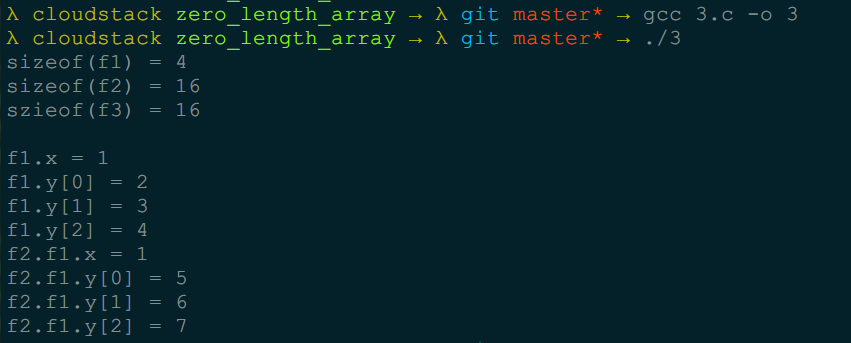# 4 0长度数组的其他特征

## 4.1 为什么0长度数组不占用存储空间

GNU C中的零长度数组

0长度数组与指针实现有什么区别呢, 为什么0长度数组不占用存储空间呢?

《 Programming Abstractions in C》（Roberts, E. S.，机械工业出版社，2004.6）82页里面说

“arr is defined to be identical to &arr”.

``````//  gdb_zero_length_array.c
#include <stdio.h>
#include <stdlib.h>

struct str
{
int len;
char s;
};

struct foo
{
struct str *a;
};

int main(void)
{
struct foo f = { NULL };

printf("sizeof(struct str) = %d\n", sizeof(struct str));

printf("before f.a->s.\n");
if(f.a->s)
{
printf("before printf f.a->s.\n");
printf(f.a->s);
printf("before printf f.a->s.\n");
}

return EXIT_SUCCESS;
}``````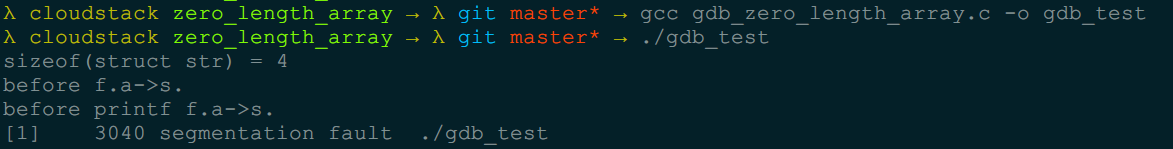``````//  gdb_pzero_length_array.c
#include <stdio.h>
#include <stdlib.h>

struct str
{
int len;
char *s;
};

struct foo
{
struct str *a;
};

int main(void)
{
struct foo f = { NULL };

printf("sizeof(struct str) = %d\n", sizeof(struct str));

printf("before f.a->s.\n");

if (f.a->s)
{
printf("before printf f.a->s.\n");
printf(f.a->s);
printf("before printf f.a->s.\n");
}

return EXIT_SUCCESS;
}``````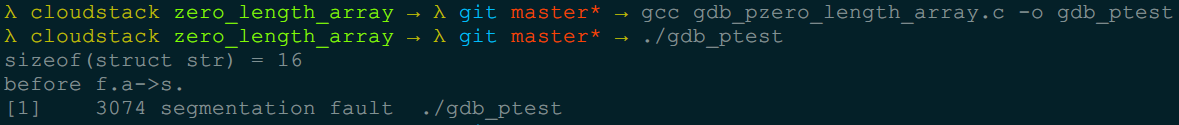``````gcc -S gdb_zero_length_array.c -o gdb_test.s
gcc -S gdb_pzero_length_array.c -o gdb_ptest
diff gdb_test.s gdb_ptest.s

1c1
<   .file   "gdb_zero_length_array.c"
---
>   .file   "gdb_pzero_length_array.c"
23c23
<   movl    \$4, %esi
---
>   movl    \$16, %esi
30c30
---
>   movq    8(%rax), %rax
36c36
---
>   movq    8(%rax), %rax``````
``````#    printf("sizeof(struct str) = %d\n", sizeof(struct str));
23c23
<   movl    \$4, %esi    #printf("sizeof(struct str) = %d\n", sizeof(struct str));
---
>   movl    \$16, %esi  #printf("sizeof(struct str) = %d\n", sizeof(struct str));``````

``````f.a->s
30c30/36c36
---
>   movq    8(%rax), %rax``````

• 对于 `char s` 来说, 汇编代码用了 `addq` 指令, addq \$4, %rax

• 对于 `char*s` 来说，汇编代码用了 `movq` 指令, `movq 8(%rax), %rax`

`addq` 对 `%rax + sizeof(struct str)`, 即`str`结构的末尾即是`char s`的地址, 这一步只是拿到了其地址, 而 `movq` 则是把地址里的内容放进去, 因此有时也被翻译为`leap`指令, 参见下一列子

• 访问相对地址，程序不会crash，但是，访问一个非法的地址中的内容，程序就会crash。

``````// 4-1.c
#include <stdio.h>
#include <stdlib.h>

int main(void)
{

char *a;
printf("%p\n", a);

return EXIT_SUCCESS;
}``````
``````4-2.c
#include <stdio.h>
#include <stdlib.h>

int main(void)
{

char a;
printf("%p\n", a);

return EXIT_SUCCESS;
}``````
``````\$ diff 4-1.s 4-2.s
1c1
<       .file   "4-1.c"
---
>       .file   "4-2.c"
13c13
<       subl    \$16, %esp
---
>       subl    \$32, %esp
15c15
<       leal    16(%esp), %eax
---
>       movl    28(%esp), %eax``````
• 对于 `char a` 来说, 汇编代码用了 `leal` 指令, `leal 16(%esp), %eax`

• 对于 `char *a` 来说，汇编代码用了 `movl` 指令, `movl 28(%esp), %eax`

## 4.2 地址优化

``````// 5-1.c
#include <stdio.h>
#include <stdlib.h>

int main(void)
{

char a;
printf("%p\n", a);

char b;
printf("%p\n", b);

return EXIT_SUCCESS;
}``````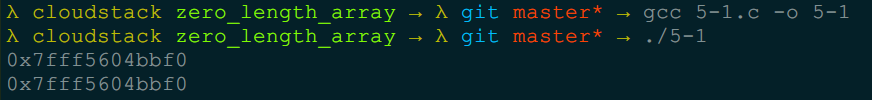``````//  5-2.c
#include <stdio.h>
#include <stdlib.h>

int main(void)
{

const char *a = "Hello";
printf("%p\n", a);

const char *b = "Hello";
printf("%p\n", b);

const char c[] = "Hello";
printf("%p\n", c);

return EXIT_SUCCESS;
}``````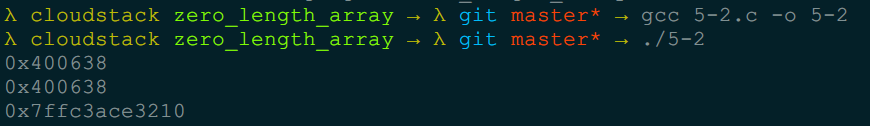# 参考

C语言中长度为0的数组

C/C++ 中的0长数组（柔性数组）

GNU C中的零长度数组

C语言变长数组data【总结】

C语言技巧之长度为0的数组

C/C++ 中长度为0的数组

GCC 中零长数组与变长数组

Multiple 0-length arrays have the same address?